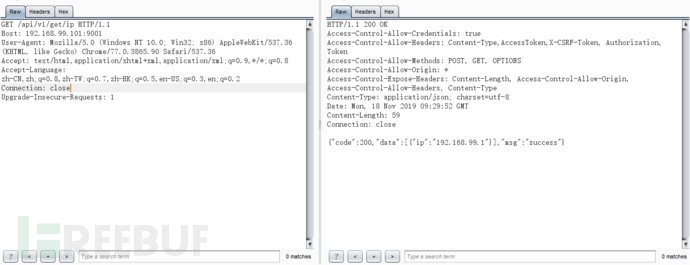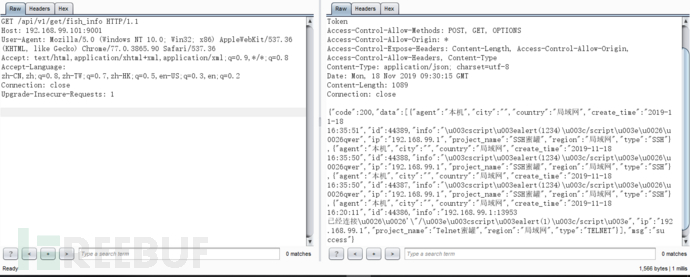# HFish蜜罐的经验

### 单节点部署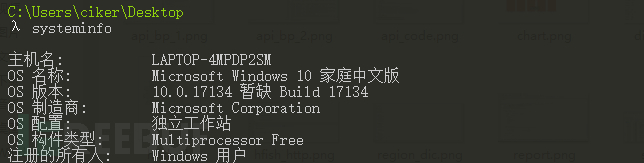eg:

``````docker run -d -p 2222:22 -p 23:23 -p 9001:9001 -e USERNAME=god -e PASSWORD=123456 --name hfish imdevops/hfish:latest
``````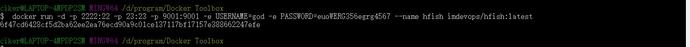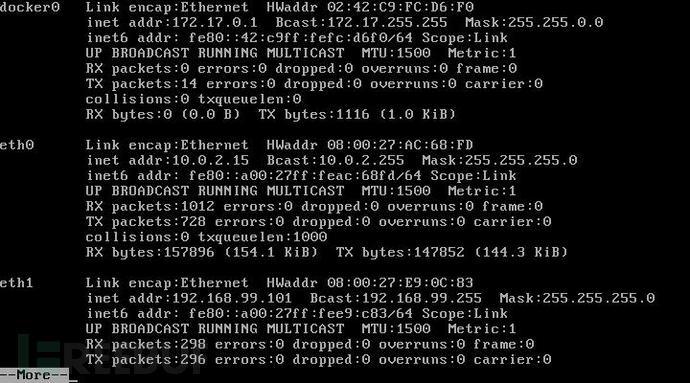### 分布式部署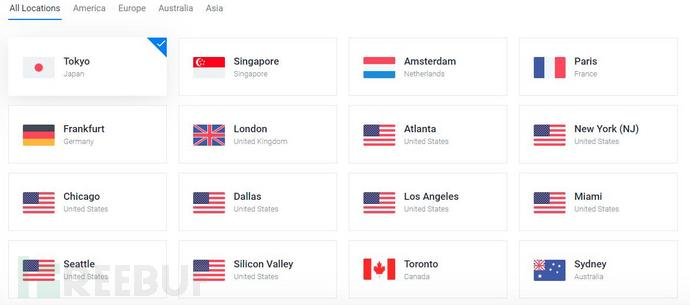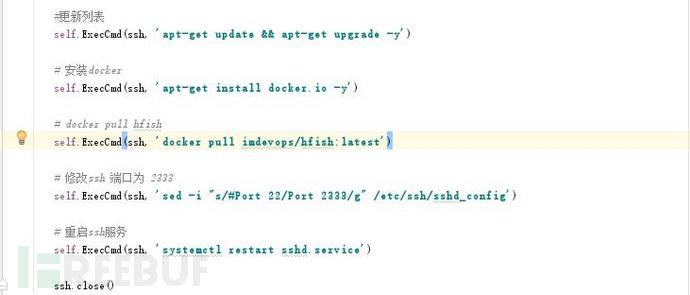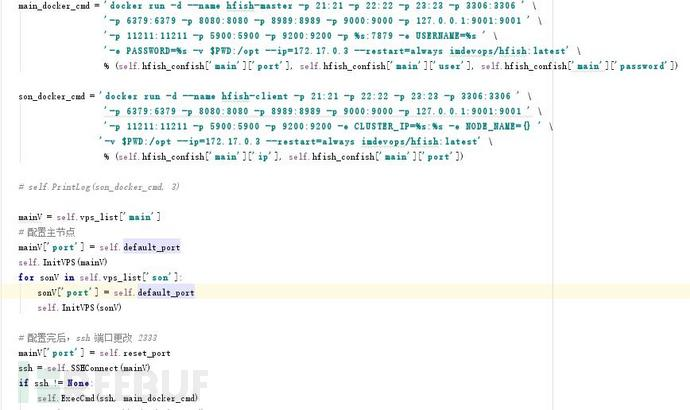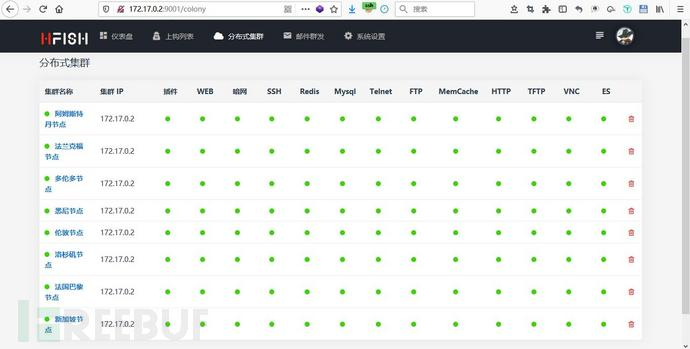## 攻击数据分析

### 攻击字典统计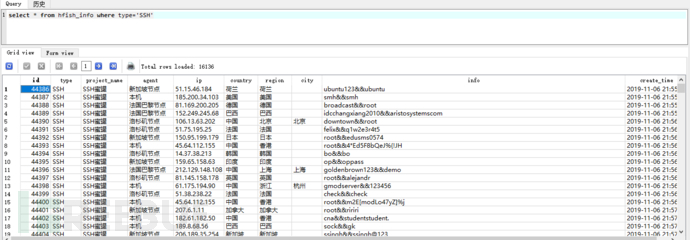``````    def __init__(self):        self.hfish_db = './file/hfish.db'        self.hfish_split = '&&'        self.sql = sqlite3.connect(self.hfish_db)        #这里需要注意一下，测试时候发现，有些字符无法用utf-8解码，所以得用bytes        self.sql.text_factory = bytes
``````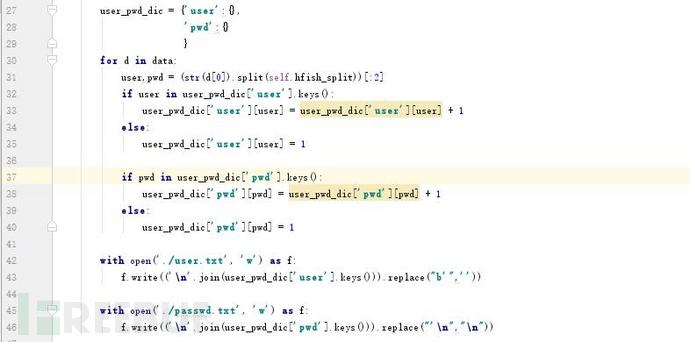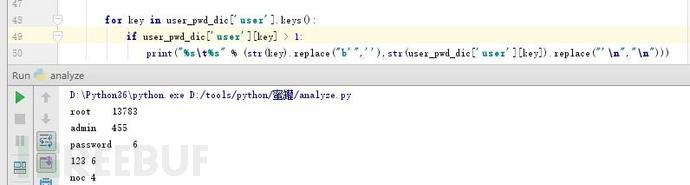``````        # 按字典值反序排序,方法一        user = sorted(user_pwd_dic['user'].items(), key=lambda x:x,reverse=True)        # 按字典值反序排序,方法二        password = list(user_pwd_dic['pwd'].items())        password.sort(key=lambda x:x,reverse=True)
``````### 字典地域性分析

``````    def RegionDic(self):        agent_sql = "select agent from hfish_info"        data = self.sql.execute(agent_sql).fetchall()        agents = []        for agent in list(set(data)):            agents.append(agent.decode('utf-8'))​        agents_dic = {}        for agent in agents:            agents_dic[agent] = {}            data = self.sql.execute(dic_sql_tpl.format(agent)).fetchall()            for d in data:                if d in agents_dic[agent].keys():                    agents_dic[agent][d] = agents_dic[agent][d] + 1                else:                    agents_dic[agent][d] = 1​            agents_dic[agent] = sorted(agents_dic[agent].items(), key=lambda x:x,reverse=True)​        for agent in agents:            print(agent)            for i in range(5):                #这里不直接用decode utf-8 是因为捕获到的一些字符无法用utf-8解码，这个在上一节连接数据库里面有提到。                print("%s\t\t%s"%(str(agents_dic[agent][i]).replace("b'",'').replace("'",''), agents_dic[agent][i]))            print()
````````````            bar = (Bar(options.InitOpts(width='1000px', height='500px'))                   .add_xaxis(u_p_dic)                   .add_yaxis(agent, u_p_count)                   .set_global_opts(                title_opts=options.TitleOpts(title=("%s统计"%agent))                    )                   )            tab.add(bar, agent)        tab.render()
``````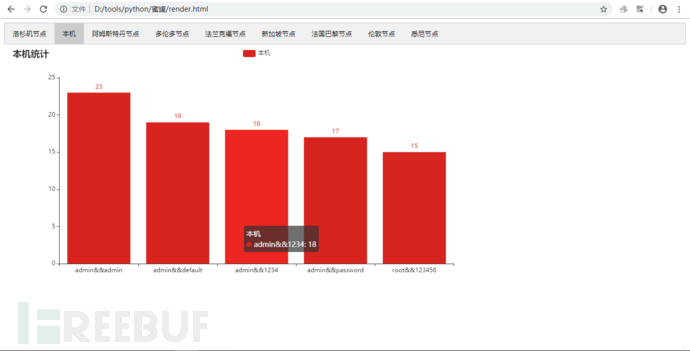## 蜜罐检测

### 基于流量识别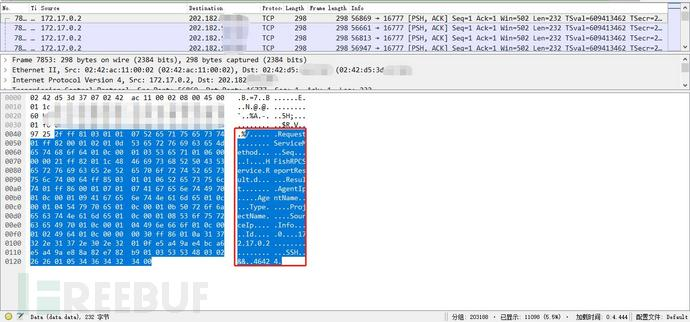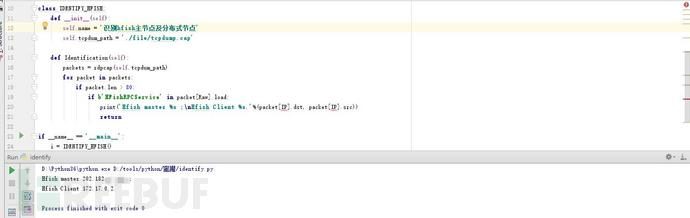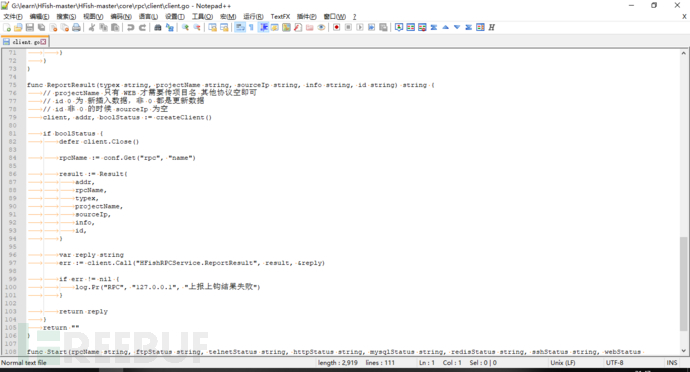### 基于高交互蜜罐返回内容识别

hfish 高交互式蜜罐是根据特征字符回显，匹配的规则有限，比如常见的读取 passwd 通常使用 cat /etc/passwd ，在SSH规则里有相应的规则``````cat /etc/passwd &
``````

``````tail /etc/passwd
``````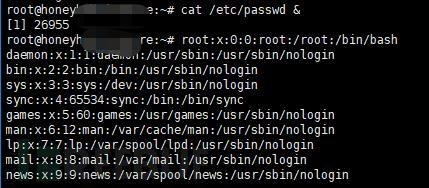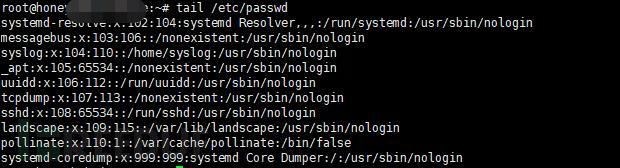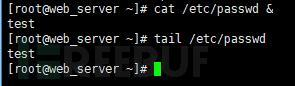### 基于管理端口返回内容识别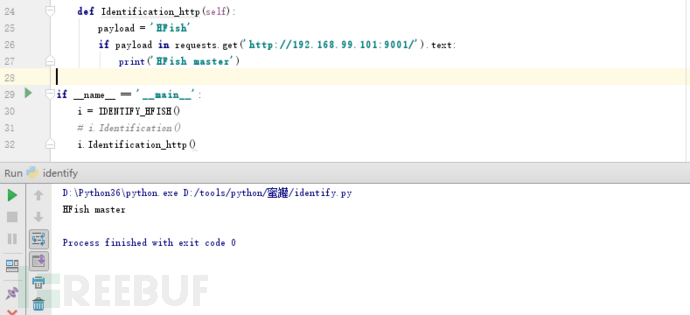### 非授权接口获取信息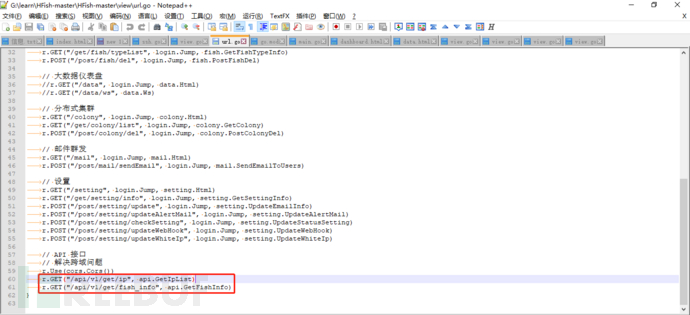``````http://ip:port/api/v1/get/iphttp://ip:port/api/v1/get/fish_info
``````